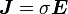# Current density at the surface of a magnetised material

## Homework Statement

Write down an expression for the current density per unit length flowing at the surface of a magnetised material.

## The Attempt at a SolutionAny ideas?

Last edited by a moderator:

diazona
Homework Helper
E is the electric field, but there's no electric field in this problem, so that equation can't apply, can it?

It sounds to me like the problem is talking about the density of bound current. There's a simple expression for that in terms of the magnetization... you can look it up, or if you think about it you can probably come up with the relation on your own. (It might help to imagine each atom of the magnetized material as a tiny little current loop)

Cyosis
Homework Helper
You've written down Ohm's law which isn't the right equation to use. I think you're looking for $\vec{K}$, the surface current density, K is the current per unit width perpendicular to the flow?

Sorted! Its I=M X n